この節の作者: Rebecca Vederhus, Sebastian Jentschke

# From SPSS to jamovi: Linear regression¶

This comparison shows how a linear model with one predictor is conducted in SPSS and jamovi. The SPSS test follows the description in chapter 9.7 in Field (2017), especially figure 9.13, and output 9.2-9.4. It uses the data set Album Sales.sav which can be downloaded from the web page accompanying the book.
SPSS jamovi
In SPSS, the following steps activates a linear regression: `Analyze``Regression``Linear`. In jamovi, this can be done using: `Analyses``Regression````Linear Regression```.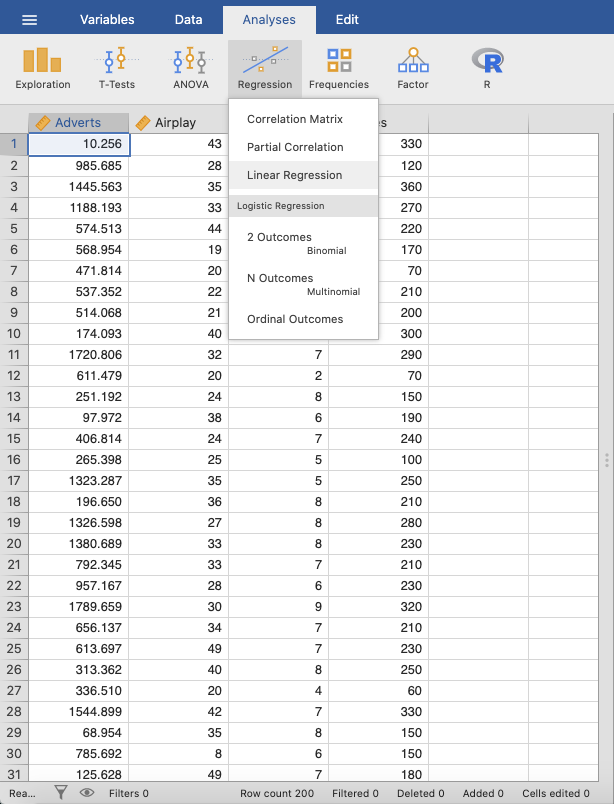In SPSS, move the variable `Sales` to the `Dependent` box and the variable `Adverts` to the `Independent(s)` box. Click `Ok`. In jamovi, move `Sales` to the `Dependent Variable` box and `Adverts` to the `Covariates` box.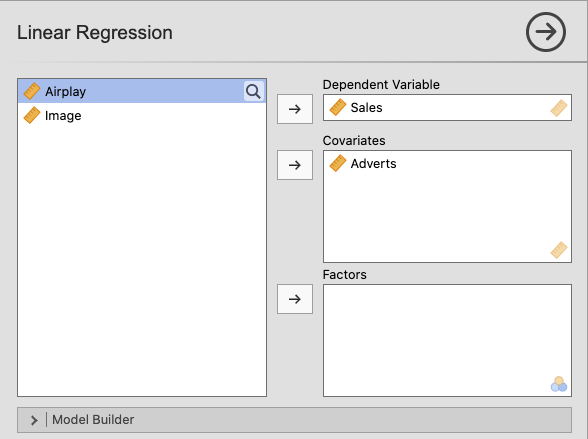Then, open the `Model Coefficients` window and press `ANOVA test` and `Standardized estimate`.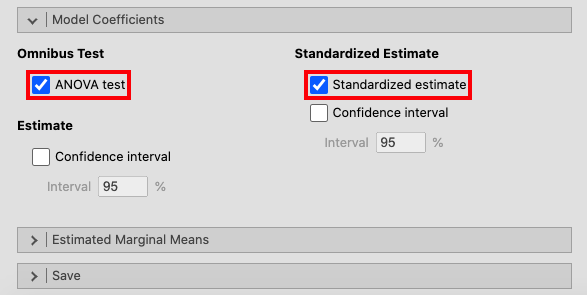In the window called `Model Fit`, click `R`, `R²` and `Adjusted R²`.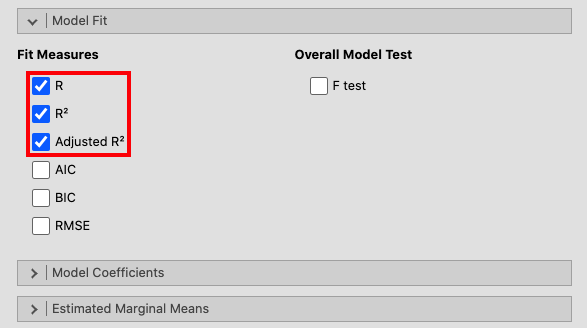Field (2017) recommends activating a bootstrapping for this analysis, but since this is not an alternative in jamovi it has been left out.
The results in SPSS and jamovi are essentially the same. However, jamovi does not display `Std. Error of the Estimate` and the total `Sum of Squares` in the ANOVA output.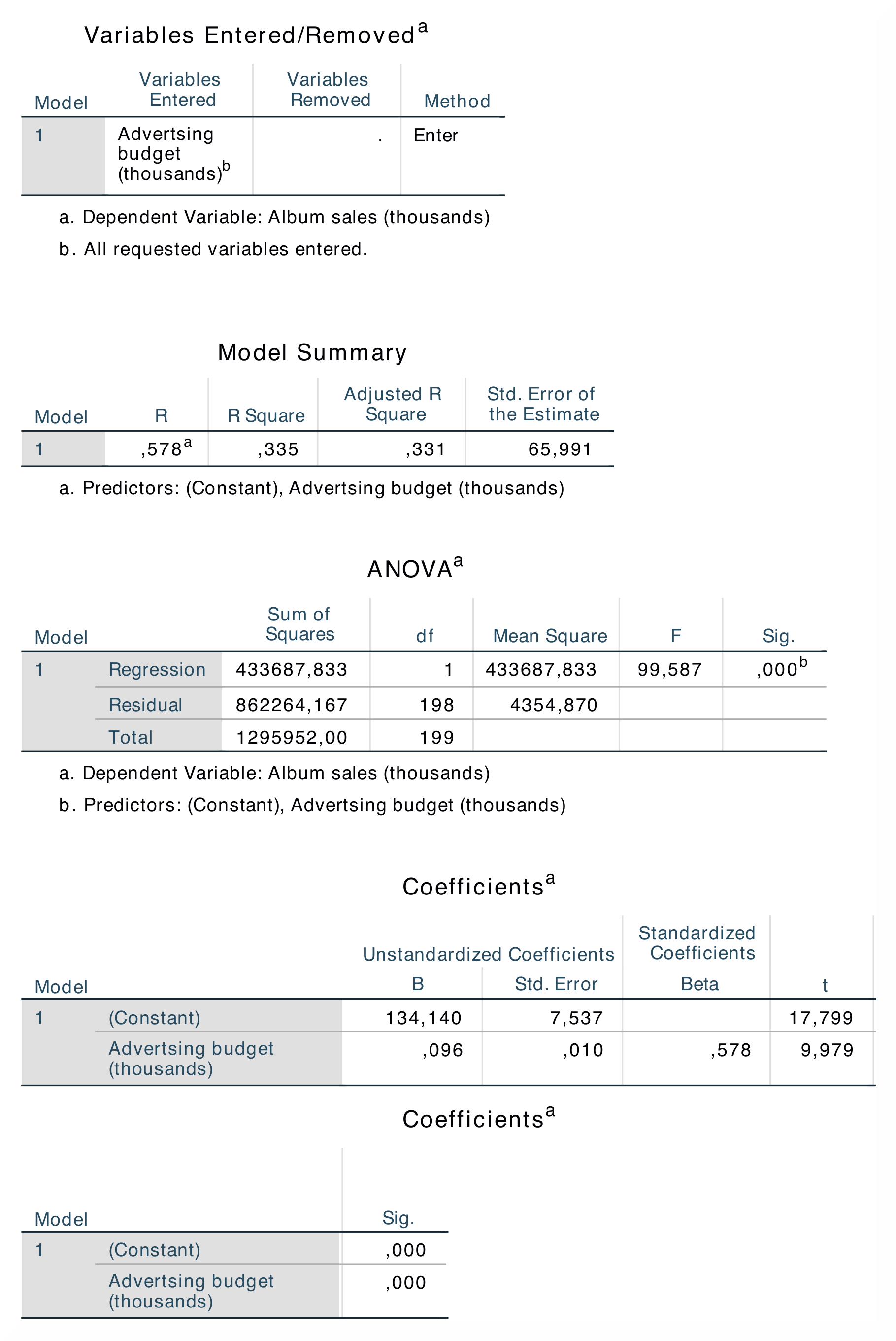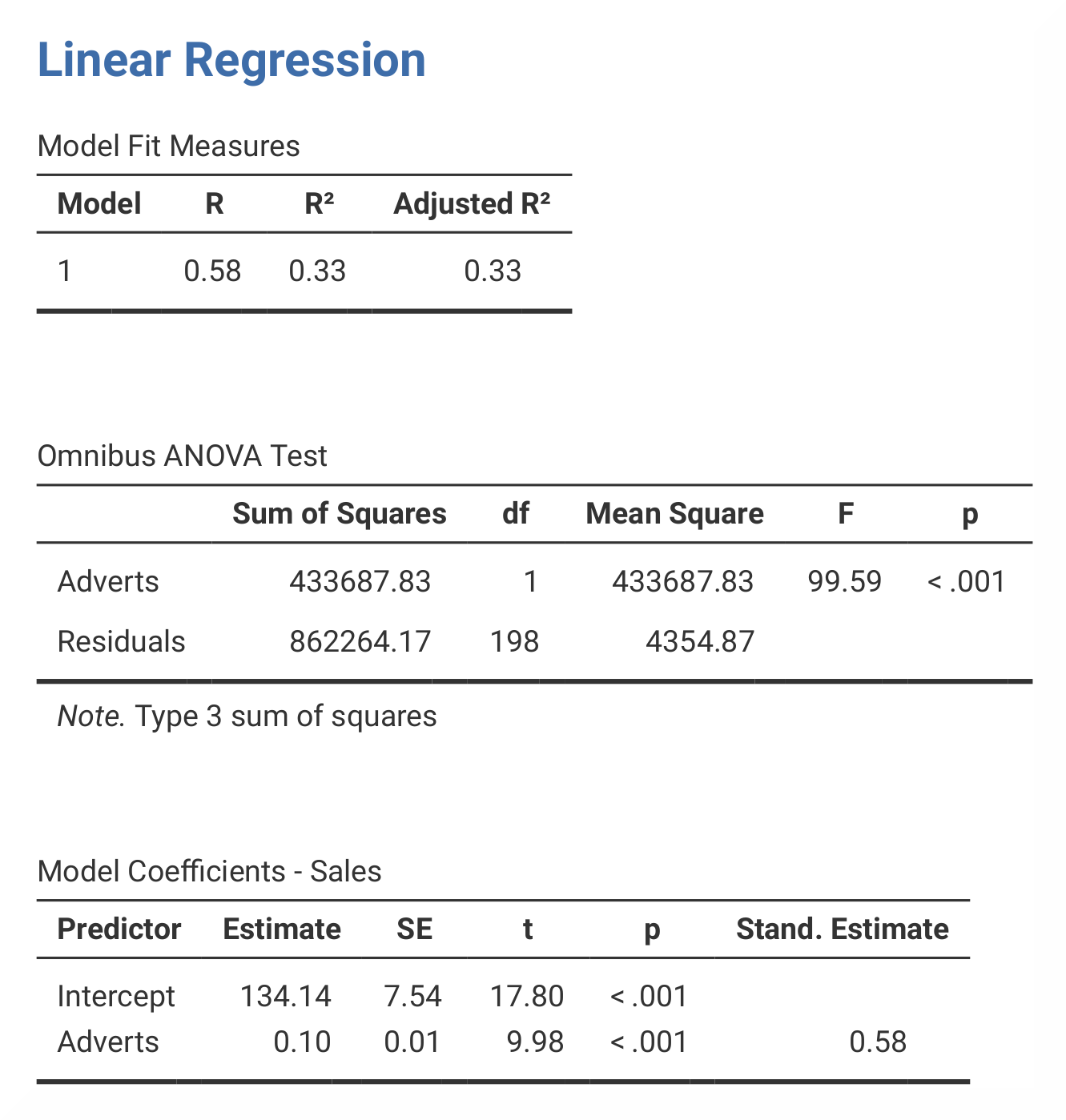The table Model Summary shows the value of R (= .578) and (= .335) for model. The most important part of the ANOVA table is the value of F (= 99.587), and its significance p (< .001). This shows that this model this model predicts album sales significantly. The Coefficients table shows the beta values and significance of these values. In jamovi, R and are found in the table called Model Fit Measures. The results from the ANOVA is found in the table called Omnibus ANOVA Test, and the beta values are found in the table Model Coefficients – Sales. In jamovi, the beta value is found in the section called Stand. Estimate, which is located at the far right of the table. As this analysis only has one predictor, the R value is the correlation between advertising and album sales. Furthermore, the value of indicates that money spent on advertising can account for 33.5% of the variance in sales of albums.
The numerical values for the statistics obtained from SPSS and jamovi are identical: R = 0.58, = 0.33, F = 99.59, p < .001; β = 0.58, p < .001.
If you wish to replicate those analyses using syntax, you can use the commands below (in jamovi, just copy to code below to Rj). Alternatively, you can download the SPSS output files and the jamovi files with the analyses from below the syntax.
```REGRESSION
/MISSING LISTWISE
/STATISTICS COEFF OUTS R ANOVA
/CRITERIA=PIN(.05) POUT(.10)
/NOORIGIN
/DEPENDENT Sales
```
```jmv::linReg(
data = data,
dep = Sales,